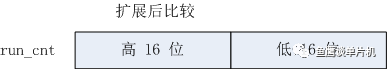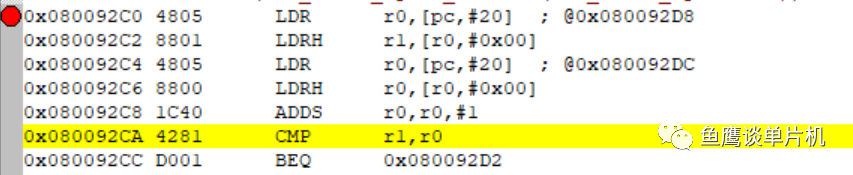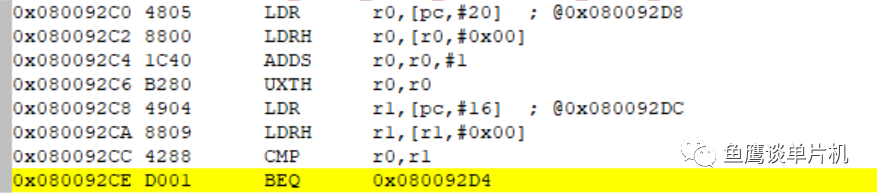• uint16_t run_cnt, run_cnt_next;

• void function1()

• {

•   do something ;

•   run_cnt++; // 自加，表示该函数已执行

• }

• int main()

• {

•   while(1)

•   {

•     function1();

•     if(run_cnt != run_cnt_next + 1) // 判断两个变量是否匹配

•     {

•       do error some thing

•     }

•     run_cnt_next++; // 这个位置也自加，表示这里已执行

•   }

• }

• if(run_cnt != run_cnt_next + 1) // 判断两个变量是否匹配

• {

•       do error some thing

• }1、常量默认为 int 型（但不一定是 32 bit ，和内核和编译器有关，上面的 +1 就是 int 型）

2、整型提升（详细可网上查找）if((uint16_t)run_cnt != (uint16_t)(run_cnt_next + 1)) // 强制转化为 16 位比较

{

do error some thing

}*博客内容为网友个人发布，仅代表博主个人观点，如有侵权请联系工作人员删除。